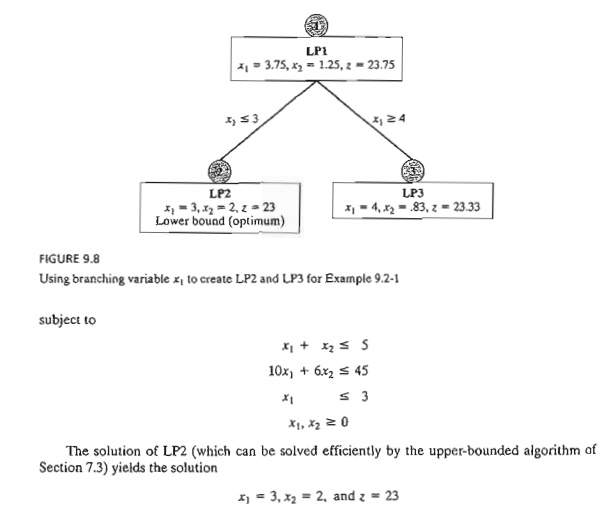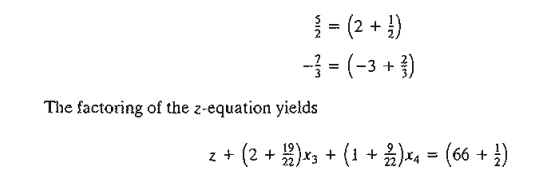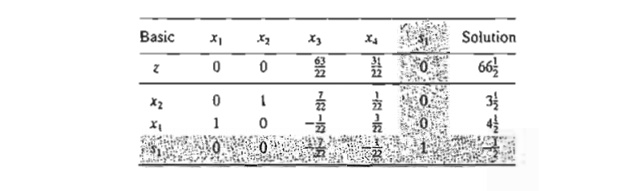Home | | Resource Management Techniques | Integer Programming Algorithms

# Integer Programming Algorithms

The ILP algorithms are based on exploiting the tremendous computational success of LP. The strategy of these algorithms involves three steps.

INTEGER PROGRAMMING ALGORITHMS

The ILP algorithms are based on exploiting the tremendous computational success of LP. The strategy of these algorithms involves three steps.

Step 1. Relax the solution space of the ILP by deleting the integer restriction on all integer variables and replacing any binary variable y with the continuous range 0 ≤ Y1. The result of the relaxation is a regular LP

Step 2. Solve the LP, and identify its continuous optimum.

Step 3. Starting from the continuous optimum point, add special constraints that iteratively modify the LP solution space in a manner that will eventually render an optimum extreme point satisfying the integer requirements.

Two general methods have been developed for generating the special constraints in step 3.

1.  Branch-and-bound (B&B) method

2.  Cutting-plane method

Although neither method is consistently effective computationally, experience shows that the B&B method is far more successful than the cutting-plane method. This point is discussed further in this chapter.

1. Branch-and-Bound (B&B) Algorithm

The first B&B algorithm was developed in 1960 by A. Land and G. Doig for the general mixed and pure ILP problem. Later, in 1965, E. Balas developed the additive algorithm for solving ILP problems with pure binary (zero or one) variable. The additive algorithm's computations were so simple (mainly addition and subtraction) that it was hailed as a possible breakthrough in the solution of general ILP. Unfortunately, it failed to produce the desired computational advantages. Moreover, the algorithm, which initially appeared unrelated to the B&B technique, was shown to be but a special case of the general Land and Doig algorithm.

This section will present the general Land-Doig B&B algorithm only. A numeric example is used to explain the details.

Example 9.2-1The lattice points (dots) in Figure 9.6 define the ILP solution space. The associated continuous LPI problem at node 1 (shaded area) is defined from ILP by removing the integer restrictions. The optimum solution of LPI is x1 = 3.75, x2 = 1.25, and z = 23.75.

Because the optimum LPI solution does not satisfy the integer requirements, the B&B algorithm modifies the solution space in a manner that eventually identifies the ILP optimum. First, we select one of the integer variables whose optimum value at LPI is not integer. Selecting xl (= 3.75) arbitrarily, the region 3 < xl < 4 of the LPI solution space contains no integer values of x1, and thus can be eliminated as nonpromising. This is equivalent to replacing the original LPI with two new LPs:Figure 9.7 depicts the LP2 and LP3 spaces. The two spaces combined contain the same feasible integer points as the original ILP, which means that, from the standpoint of the integer solution, dealing with LP2 and LP3 is the same as dealing with the original LPl; no information is lost.

If we intelligently continue to remove the regions that do not include integer solutions (e.g., < xl < 4 at LPl) by imposing the appropriate constraints, we will eventually produce LPs whose optimum extreme points satisfy the integer restrictions. In effect, we will be solving the ILP by dealing with a sequence of (continuous) LPs.

The new restrictions, x1 ≤ 3 and x1  4, are mutually exclusive, so that LP2 and LP3 at nodes 2 and 3 must be dealt with as separate LPs, as Figure 9.8 shows. This dichotomization gives rise to the concept of branching in the B&B algorithm. In this case, x1 is called the branching variable.

The optimum ILP lies in either LP2 or LP3. Hence, both subproblems must be examined. We arbitrarily examine LP2 (associated with x1 ≤3) first:The LP2 solution satisfies the integer requirements for xl and x2. Hence, LP2 is said to be fathomed, meaning that it need not be investigated any further because it cannot yield any beller ILP solution.

We cannot at this point say that the integer solution obtained from LP2 is optimum for the original problem, because LP3 may yield a better integer solution with a higher value of z. All we can say is that z = 23 is a lower bound on the optimum (maximum) objective value of the original ILP. This means that any unexamined subproblem that cannot yield a better objective value than the lower bound must be discarded as nonpromising. If an unexamined subproblem produces a better integer solution, then the lower bound must be updated accordingly.

Given the lower bound z = 23, we examine LP3 (the only remaining unexamined subproblem at this point). Because optimum z = 23.75 at LPI and all the coefficients of the objective function happen to be integers, it is impossible that LP3 (which is more restrictive than LPl) will produce a better integer solution with z > 23. As a result, we discard LP3 and conclude that it has been fathomed.

The B&B algorithm is now complete because both LP2 and LP3 have been examined and fathomed (the first for producing an integer solution and the second for failing to produce a better integer solution). We thus conclude that the optimum ILP solution is the one associated with the lower bound-namely, x1 = 3, x2, and z = 23.

Two questions remain unanswered regarding the procedure.

a)     At LPI, could we have selected x2 as the branching variable in place of xl?

b)    When selecting the next subproblem to be examined, could we have solved LP3 first in-stead of LP2?

The answer to both questions is "yes," but ensuing computations could differ dramatically. Figure 9.9 demonstrates this point. Suppose that we examine LP3 first (instead of LP2 as we did in Figure 9.8). The solution is xI = 4, x2 = .83, and z = 23.33 (verify!). Because x2 (= .83) is noninteger, LP3 is examined further by creating subproblems LP4 and LPS using the branches x2 ≤ 0 and x2  1, respectively. This means thatWe now have three "dangling" subproblems to be examined: LP2, LP4, and LP5. Suppose that we arbitrarily examine LP5 first. LP5 has no solution, and hence it is fathomed. Next, let us examine LP4. The optimum solution is xl = 4.5, x2 = 0, and z = 22.5. The noninteger value of x1 leads to the two branches x 4 and Xl ≥ 5, and the creation of subproblems LP6 and LP7 from LP4.Now, subproblems LP2, LP6, and LP7 remain unexamined. Selecting LP7 for examination, the problem has no feasible solution, and thus is fathomed. Next, we select LP6. The problem yields the first integer solution (xl = 4, x2 = 0, z = 20), and thus provides the first lower bound(= 20) on the optimum ILP objective value. We are now left with subproblem LP2, which yields a better integer solution (x1 = 3, x2 = 2, z = 23). Thus, the lower bound is updated from z = 20 to = 23. At this point, all the subproblems have been fathomed (examined) and the optimum solution is the one associated with the most up-to-date lower bound-namely, xl = 3, x2 = 2, and = 23.

The solution sequence in Figure 9.9 (LPl --> LP3 --> LP5 --> LP4 --> LP7 --> LP6 --> LP2) is a worst-case scenario that, nevertheless, may well occur in practice. In Figure 9.8, we were lucky to "stumble" upon a good lower bound at the very first subproblem we examined (LP2), thus allowing us to fathom LP3 without investigating it. In essence, we compieted the procedure by solving a total of two LPs. In Figure 9.9, the story is different: We needed to solve seven LPs before the B&B algo-rithm could be terminated.

Remarks. The example points to a principal weakness in the B&B algorithm: Given multiple choices, how do we select the next subproblem and its branching variable? Although there are heuristics for enhancing the ability of B&B to "foresee" which branch can lead to an improved ILP solution (see Taha, 1975, pp. 154-171), solid theory with consistent results does not exist, and herein lies the difficulty that plagues computations in ILP. Indeed, Problem 7, Set 9.2a, demonstrates the bizarre behavior of the B&B algorithm in investigating over 25,000 LPs before optimality is verified, even though the problem is quite small (16 binary variables and 1 constraint). Unfortunately, to date, and after more than four decades of research coupled with tremendous advances in computing power, available ILP codes (commercial and academic alike) are not to-tally reliable, in the sense that they may not find the optimum ILP solution regardless of how long they execute on the computer. What is even more frustrating is that this behavior can apply just the same to some relatively small problems.

AMPL Moment

AMPL can be used interactively to generate the B&B search tree. The following table shows the sequence of commands needed to generate the tree of Example 9.2-1 (Figure 9.9) starting with the continuous LPO. AMPL model (file ampIEx9.2-1.txt) has two variables xl and x2 and two constraints c0 and cl. You will find it helpful to synchronize the AMPL commands with the branches in Figure 9.9.Solver Moment

Solver can be used to obtain the solution of the different subproblems by using the add/change/delete options in the Solver Parameters dialogue box.

Summary of the B&B Algorithm. We now summarize the B&B algorithm. Assuming a maximization problem, set an initial lower bound z = - ¥ on the optimum objective value of ILP. Set i = 0.

Step 1. (Fathoming/bounding). Select LPi, the next subproblem to be examined. Solve LPi, and attempt to fathom it using one of three conditions:

a)   The optimal z-value of LPi cannot yield a better objective value than the current lower bound.

b)   LPi yields a better feasible integer solution than the current lower bound.

c)     LPi has no feasible solution.

Two cases will arise.

a)     If LPi is fathomed and a better solution is found, update the lower bound. If all subproblems have been fathomed, stop; the optimum ILP is associated with the current finite lower bound. If no finite lower bound exists, the problem has no feasible solution. Else, set i = i + 1, and repeat step 1.

b)    If LPi is not fathomed, go to step 2 for branching.

Step 2. (Branching). Select one of the integer variables xj, whose optimum value xj* in the LPi solution is not integer. Eliminate the region(where [v] defines the largest integer ≤ v) by creating two LP subproblems that correspond toThe given steps apply to maximization problems. For minimization, we replace the lower bound with an upper bound (whose initial value is z = +¥).

The B&B algorithm can be extended directly to mixed problems (in which only some of the variables are integer). If a variable is continuous, we simply never select it as a branching variable. A feasible subproblem provides a new bound on the objective value if the values of the discrete variables are integer and the objective value is improved relative to the current bound.

2. Cutting-Plane Algorithm

As in the B&B algorithm, the cutting-plane algorithm also starts at the continuous optimum LP solution. Special constraints (called cuts) are added to the solution space in a manner that renders an integer optimum extreme point. In Example 9.2-2, we first demonstrate graphically how cuts are used to produce an integer solution and then implement the idea algebraically.

Example 9.2-2

Consider the following ILl'.The cutting-plane algorithm modifies the solution space by adding cuts that produce an optimum integer extreme point. Figure 9.10 gives an example of two such cuts.

Initially, we start with the continuous LP optimum z = 66(1/2), x1  = 4(1/2), x2  =  3(4/7). Next, we add cut I, which produces the (continuous) LP optimum solution z = 62, x1 = 4(4/7), x2 = 3. Then, we add cut II, which, together with cut I and the original constraints, produces the LP optimum = 58, xl = 4, x2 = 3. The last solution is all integer, as desired.

The added cuts do not eliminate any of the original feasible integer points, but must pass through at least one feasible or infeasible integer point. These are basic requirements of any cut.

FIGURE 9.10

Illustration of the use of cuts in ILPIt is purely accidental that a 2-variable problem used exactly 2 cuts to reach the optimum integer solution. In general, the number of cuts, though finite, is independent of the size of the problem, in the sense that a problem with a small number of variables and constraints may re-quire more cuts than a larger problem.

Next, we use the same example to show how the cuts are constructed and implemented algebraically.

Given the slacks x3 and x4 for constraints 1 and 2, the optimum LP tableau is given asThe optimum continuous solution is z = 66(1/2), x1= 4(1/2), x2 = 3(1/2), x3 = 0, x4 = 0. The cut is developed under the assumption that all the variables (including the slacks x3 and x4) are integer. Note also that because all the original objective coefficients are integer in this example, the value of z is integer as well.

The information in the optimum tableau can be written explicitly asA constraint equation can be used as a source row for generating a cut, provided its right-hand side is fractional. We also note that the z-equation can be used as a source row because z happens to be integer in this example. We will demonstrate how a cut is generated from each of these source rows, starting with the z-equation.

First, we factor out all the noninteger coefficients of the equation into an integer value and a fractional component, provided that the resulting fractional component is strictly positive. For example,Moving all the integer components to the left-hand side and all the fractional components to the right-hand side, we getBecause x3 and x4 are nonnegative and all fractions are originally strictly positive, the right-hand side must satisfy the following inequality:This result is justified because an integer value ≤ 1/2 must necessarily be ≤ 0 .

The last inequality is the desired cut and it represents a necessary (but not sufficient) condition for obtaining an integer solution. It is also referred to as the fractional cut because all its co-efficients are fractions.

Because x3 = x4 = 0 in the optimum continuous LP tableau given above, the current continu-ous solution violates the cut (because it yields 1/2 ≤ 0). Thus, if we add this cut to the optimum tableau, the resulting optimum extreme point moves the solution toward satisfying the integer requirements.

Before showing how a cut is implemented in the optimal tableau, we will demonstrate how cuts can also be constructed from the constraint equations. Consider the x1-row:Anyone of three cuts given above can be used in the first iteration of the cutting-plane a1gorithm. It is not necessary to generate all three cuts before selecting one.

Arbitrarily selecting the cut generated from the x2-row, we can write it in equation form asThis constraint is added to the LP optimum tableau as follows:The tableau is optimal but infeasible. We apply the dual simplex method (Section 4.4.1) to recover feasibility, which yieldsThe last solution is still noninteger in xl and x3. Let us arbitrarily select xl as the next source row-that is,The dual simplex method yields the following tableau:The optimum solution (xl = 4, x2 = 3, z = 58) is all integer. It is not accidental that all the coefficients of the last tableau are integers, a property of the implementation of the fractional cut.

Remarks. It is important to point out that the fractional cut assumes that all the variables, including slack and surplus, are integer. This means that the cut deals with pure integer problems only. The importance of this assumption is illustrated by an example.

Consider the constraintFrom the standpoint of solving the associated ILP, the constraint is treated as an equation by using the nonnegative slack sl-that is,The application of the fractional cut assumes that the constraint has a feasible integer solution in all xlx2, and sl. However, the equation above will have a feasible integer solution in xl and x2 only if x1 is noninteger. This means that the cutting-plane algorithm will show that the problem has no feasible integer solution, even though the variables of concern, xl and x2, can assume feasible integer values.

There are two ways to remedy this situation.

1. Multiply the entire constraint by a proper constant to remove all the fractions. For example, multiplying the constraint above by 6, we getAny integer solution of x1 and x2 automatically yields integer slack. However, this type of conversion is appropriate for only simple constraints, because the magnitudes of the integer coefficients may become excessively large in some cases.

2. Use a special cut, called the mixed cut, which allows only a subset of variables to assume integer values, with all the other variables (including slack and surplus) remaining continuous. The details of this cut will not be presented in this chapter (see Taha, 1975, pp. 198-202).

Study Material, Lecturing Notes, Assignment, Reference, Wiki description explanation, brief detail
Operations Research: An Introduction : Integer Linear Programming : Integer Programming Algorithms |